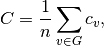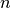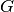Warning

This documents an unmaintained version of NetworkX. Please upgrade to a maintained version and see the current NetworkX documentation.

# average_clustering¶

average_clustering(G, nodes=None, weight=None, count_zeros=True)[source]

Compute the average clustering coefficient for the graph G.

The clustering coefficient for the graph is the average,whereis the number of nodes in.

Parameters: G (graph) – nodes (container of nodes, optional (default=all nodes in G)) – Compute average clustering for nodes in this container. weight (string or None, optional (default=None)) – The edge attribute that holds the numerical value used as a weight. If None, then each edge has weight 1. count_zeros (bool) – If False include only the nodes with nonzero clustering in the average. avg – Average clustering float

Examples

>>> G=nx.complete_graph(5)
>>> print(nx.average_clustering(G))
1.0


Notes

This is a space saving routine; it might be faster to use the clustering function to get a list and then take the average.

Self loops are ignored.

References

  Generalizations of the clustering coefficient to weighted complex networks by J. Saramäki, M. Kivelä, J.-P. Onnela, K. Kaski, and J. Kertész, Physical Review E, 75 027105 (2007). http://jponnela.com/web_documents/a9.pdf
  Marcus Kaiser, Mean clustering coefficients: the role of isolated nodes and leafs on clustering measures for small-world networks. http://arxiv.org/abs/0802.2512